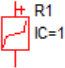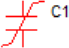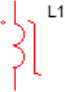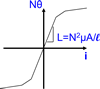Handout D: SIMPLIS PWL R, L, C

The table below illustrates how SIMPLIS represents PWL resistors, PWL capacitors, and PWL inductors as a set of PWL straight-line segments. A PWL resistor is piecewise linear in the current versus the voltage plane. The slope of this curve is the conductance. SIMPLIS automatically extends the left-most and right-most PWL segment to negative and positive infinity, respectively.

PWL capacitors are defined on the charge versus voltage plane where the slope is capacitance. PWL inductors are defined on the flux-linkage versus current plane, where the slope is inductance. The units of flux linkage are Weber-Turns or volt-seconds.

 PWL Resistor PWL Capacitor PWL Inductorx-value ⇒ Voltage x-value ⇒ Voltage x-value ⇒ Current y-value ⇒ Current y-value ⇒ Charge y-value ⇒ Flux Linkage Slope=1/Resistance Slope=Capacitance Slope=Inductance